# Intermediate Geometry : How to find the length of the side of a parallelogram

## Example Questions

### Example Question #31 : Parallelograms

Find the perimeter of the following box in inches: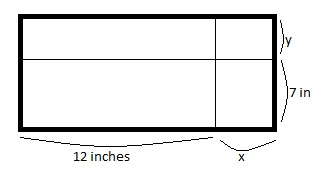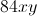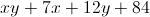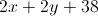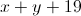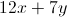Explanation:

The answer is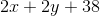You can find the perimeter by adding all of its respective sides as such: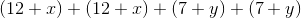Adding like terms will result inIf you chose, you multiplied the two sides to find the area.

If you chose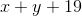, you only added two sides. Perimeter involves all 4 sides; so double the width and length.

Just remember, the width is 12 added to.  Not 12 times the side of### Example Question #32 : Parallelograms

A parallelogram has an area of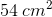. If the height is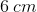, what is the length of the base?

Cannot be determined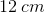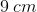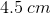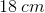Explanation:

If the area of a parallelogram is given aswith a height of, we can refer back to the equation for the area of a parallelogram: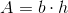, whereis height andis the length of the base.

This very quickly becomes a problem of substituting in values and finding the value of an unknown variable, in this case,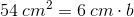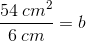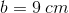### Example Question #33 : Parallelograms

A parallelogram has a base of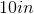and an area of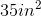. What is the height of the parallelogram?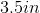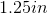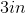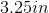Explanation:

In order to find the height of this parallelogram apply the formula: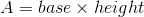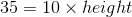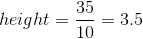### Example Question #34 : Parallelograms

A parallelogram has a height of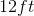and an area of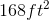. What is the length of the base of the parallelogram?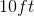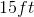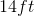Explanation:

To find the missing side of this parallelgram apply the formula:Thus, the solution is: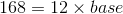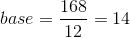### Example Question #1 : How To Find The Length Of The Side Of A Parallelogram

Given that a parallelogram has a height of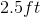and an area of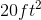. Find the base of the parallelogram.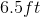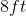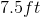Explanation:

In order to find the base of this parallelogram apply the formula: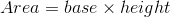Thus, the solution is: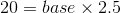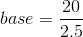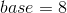### Example Question #2 : How To Find The Length Of The Side Of A Parallelogram

Given: Quadrilateralwith diagonal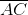;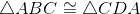.

True or false: From the information given, it follows that Quadrilateralis a parallelogram.

True

False

True

Explanation:

Corresponding parts of congruent triangles are, by definition, congruent. Thus, from the statement, it follows that: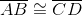and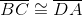Quadrilateraltherefore has two sets of congruent opposite sides. This is a sufficient condition for the quadrilateral to be a parallelogram.

### Example Question #3 : How To Find The Length Of The Side Of A Parallelogram

Quadrilateralis both a rhombus and a rectangle.

True or false: Quadrilateralmust be a square.

True

False